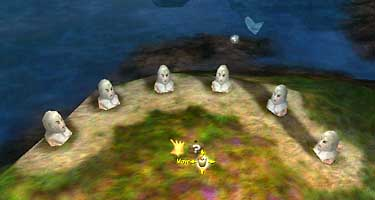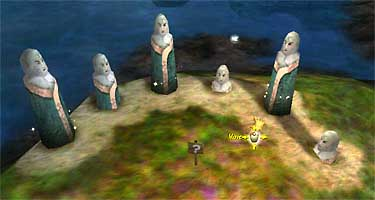# Black & White’s Totem Puzzle

One of the game Black & White’s non-silver scroll challenges on land 4 is the Japanese totem puzzle. Not the one that frees the cursed village; this one is on the coast, on a small bluff above the silver scroll fish-herding challenge. Your goal is to raise all six of the totems to their full height simultaneously. What makes this a challenge is that raising one totem can move other totems.Totem puzzle in starting configuration

I couldn’t find a solution to (or even a mention of) this puzzle on the Web, which I suppose means that either (a) it’s trivially simple, (b) no one notices it, (c) it’s so hard no one completes it, or (d) it’s too boring to remember. But having noticed it, and being of an analytic bent, I decided to find a solution better than trial and error.

Some ground rules:

• At the start, all totems are down.

• Each totem has five positions: down, 1/4 up, half up, 3/4 up, and fully up. (I call these 0, 1, 2, 3, and 4, respectively.) When a totem is fully up, its base sparkles.

By playing with the totems, I discovered several things:

• Moving totem #2 (numbered from left to right) affects no other totems.

• Totem #5 is affected by no other totems.

These two facts simplify the puzzle: you can start by fully raising totem #5, then concentrate on raising totems #1, 3, 4, and 6. When you’ve got them up, raise #2.

• The totems move by modular arithmetic, mod 5 to be specific. That is, if totem A is fully raised (position 4) and moving B would raise A another notch (+1), then A would return to the down (0) position (because 4 + 1 = 5 = 0 mod 5).

• One totem’s affect on another totem is independent of the current state of the puzzle. If raising totem X a notch also raises totem Y a notch, then this will always be the case, no matter how the other totems have affected totem Y.

• Moves are invertible: lowering a totem one notch cancels the effects of raising it one notch. Hence, moves can be undone.

• From the last two facts, it follows that moves are independent (the order of moves doesn’t matter): A followed by B gives the same results as B followed by A.

• Because moves are independent and invertible, you need move each totem only once. (Given moves A and A', which both move one totem, and B, which moves another, ABA' = AA'B, and AA' is equal to one movement of A.) The trick is to figure out how much to move each totem.

How raising a totem one position affects the totems is given in the following table. The column indicates which totem is raised one position, while the row indicates the totem affected. (For example, raising totem #6 one notch raises totem #2 three notches.) I use 0-4 notation here, leaving out the zeros for clarity.

1 2 3 4 5 6 1 2 3 totem raised totemaffected 1 1 4 1 1 3 1 1 2 1 1 1 2 1 1 A B C D E F variable

We want all six totems to be in position 4, which is fully raised. Let the amount we raise totem #1 be A, the amount we raise #2 to be B, and so on to #6, which is F. Let’s look at totem #1 (row 1). The only totems that affect it are itself (obviously) and totem #3, which moves it one notch per notch totem #3 moves. As an equation, this is A + C = 4 mod 5. [It’s mod 5 because raising a totem 5 notches is the same as if you hadn’t moved it at all, while lowering a totem to -1 is the same as raising it to 4.] Likewise, we can read across the rest of the rows to get six equations:

```  A      +  C                = 4 mod 5
4A +  B +  C           + 3F = 4 mod 5
C +  D           = 4 mod 5
2C +  D      +  F = 4 mod 5
E      = 4 mod 5
2A                +  E +  F = 4 mod 5
```

From the fifth equation we have E = 4. (This isn’t surprising, since we noted above that totem #5 is affected by no other totems.)

Now look at the first and third equations:

```  A      +  C                = 4 mod 5
C +  D           = 4 mod 5
```

These imply that A = D. Substituting A for D, and also using the fact that E = 4, our equations now look like this:

```  A      +  C                = 4 mod 5
4A +  B +  C           + 3F = 4 mod 5
C +  A           = 4 mod 5   (same as first equation, so we’ll omit it from now on)
2C +  A      +  F = 4 mod 5
E      = 4         (which we’ll also omit)
2A                     +  F = 0 mod 5   (0 because we subtracted E = 4 from both sides)
```

Subtracting the first equation from the fourth gives

```  A      +  C                = 4 mod 5
4A +  B +  C           + 3F = 4 mod 5
C           +  F = 0 mod 5
2A                     +  F = 0 mod 5
```

Subtracting twice the fourth equation from the second gives

```  A      +  C                = 4 mod 5
B +  C           +  F = 4 mod 5
C           +  F = 0 mod 5
2A                     +  F = 0 mod 5
```

Subtracting the third equation from the second reveals that B = 4. This leaves just three equations:

```  A      +  C                = 4 mod 5
C           +  F = 0 mod 5   (ii)
2A                     +  F = 0 mod 5   (iii)
```

By comparing the last two equations we find that C = 2A. That means (ii) and (iii) are the same, and we’re left with just two equations.

```  A      +  C                = 4 mod 5
C           +  F = 0 mod 5
```

Substituting C = 2A in the first equation gives 3A = 4 mod 5. The obvious solution is A = 3. This makes C = 2A mod 5 = 1, which in turn means F = 4. With B = E = 4 and D = A, we have a complete solution:

totem move...
1 Raise three notches
2 Lower one notch
3 Raise one notch
4 Lower two notches
5 Fully raise
6 Fully raiseThe penultimate moment before raising totem #6

The reward for solving the puzzle is a Spiritual Shield miracle dispenser, which is a booby prize considering the amount of analysis required; there’s already another Spiritual Shield miracle dispenser close to your temple, plus your starting tribe on that land has the miracle too. For all that work I want something better, like a Spiritual Shield extreme. Or Megablast.

Of course, your real reward is learning some algebra. Those sneaky Lionhead coders.

Last updated 25 August 2006
http://www.rdrop.com/~half/General/GameTips/bw.totem.puzzle.html
All contents ©2006 Mark L. Irons